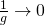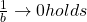# lens equation

The “Gauss lens equation,” as it is known, goes like this:where
f = focal length,
g = distance to object (measured from object side principal plane H).
b = distance to image (measured from image side principal plane H’).

It can be solved for focal length, object distance and image distance.

## We may find the focal length by this equation:## We may find the object distance by this equation:## We may find the image distance by this equation:## Interpretation of the lens equation

What can be derived from this formula?

• The focus plane contains the image of infinitely far away objects.
• When the object moves to infinity (= when g gets infinitely large), thenAnd therefore• On the object side, an object with a double focal length distance is mapped to an image with a double focus length distance on the image side.
• In other words : If we want a 1:1 mapping in distance X from the sensor you have to choose
•(but subtract the distance of the principal points from X)

• The picture is at infinity when the object distance equals the focus length.
• When an object nears to the focus length, the image distance rises. Therefore.
• For an object closer to the lens than the focal lengthholds: the image is generated on the object side (!) of the lens.
• The object and image distances are calculated from the primary planes on the object and image sides, respectively.
As a result, the object distance differs from the working distance in most cases.
The focal length is a paraxial concept. Therefore the Gauss lens equation is only valid in the paraxial region of the lens, the region whereholds. Most calculators don’t care (including ours), As a result, anytime larger viewing angles are involved, the results are essentially a “informed guess.”